Impact Factor 3.560 | CiteScore 3.1
More on impact ›

# Frontiers in Physics## ORIGINAL RESEARCH article

Front. Phys., 13 January 2021 | https://doi.org/10.3389/fphy.2020.579461

# Efficient Numerical Solution of the EMI Model Representing the Extracellular Space (E), Cell Membrane (M) and Intracellular Space (I) of a Collection of Cardiac Cells

• 1Simula Research Laboratory, Lysaker, Norway
• 2Department of Informatics, University of Oslo, Oslo, Norway

The EMI model represents excitable cells in a more accurate manner than traditional homogenized models at the price of increased computational complexity. The increased complexity of solving the EMI model stems from a significant increase in the number of computational nodes and from the form of the linear systems that need to be solved. Here, we will show that the latter problem can be solved by careful use of operator splitting of the spatially coupled equations. By using this method, the linear systems can be broken into sub-problems that are of the classical type of linear, elliptic boundary value problems. Therefore, the vast collection of methods for solving linear, elliptic partial differential equations can be used. We demonstrate that this enables us to solve the systems using shared-memory parallel computers. The computing time scales perfectly with the number of physical cells. For a collection of 512 $×$ 256 cells, we solved linear systems with about $2.5×{10}^{8}$ unknows. Since the computational effort scales linearly with the number of physical cells, we believe that larger computers can be used to simulate millions of excitable cells and thus allow careful analysis of physiological systems of great importance.

## 1. Introduction

Traditionally, numerical simulations of excitable tissue have been performed using homogenized mathematical models. For cardiac tissue, the bidomain model and the associated monodomain model have been very popular and provided important insights into the electrochemical processes underpinning every heartbeat. Introductions to these models can be found in, e.g., Refs. 1 and 2 and numerical methods used to solved the models are presented in numerous papers; see, e.g., Refs. 37. In the homogenized models, the extracellular space, the cell membrane and the intracellular space all exist everywhere in the computational domain. This approach has proven to be very useful in studying effects on a relatively large length scale (millimeters), but has obvious limitations when the length scale approaches the size of a cell (micrometers). The main reason for this is that the cell itself is missing in the homogenized models.

As an alternative to the homogenized models, we have recently presented and applied a cell-based model; see, e.g., Refs. 810. In this model, the extracellular domain (E), the cell membrane (M) and the intracellular domain (I) are all present in the mathematical model and it is therefore referred to as the EMI model. The EMI model is motivated by earlier work where homogenization has been avoided; see, e.g., Refs. 1119.

The obvious advantage of the EMI approach over the homogenized counterparts is that the cell itself is present in the model. This enables the modeler to change cell membrane properties locally (e.g., varying ion channel densities along the cell membrane) which may be of importance for the conduction velocity; see, e.g., Refs. 9, 20 and 21. Furthermore, the presence of the cell allows for study of the effect of changes to the cell size and shape which may be of great importance; see, e.g., Refs. 2225. For instance, the T-tubule system can only be represented in an averaged manner in the classical bidomain/monodomain models, but in the EMI model it would be possible to represent the T-tubule system explicitly albeit at the cost of handling complex geometries.

On the other hand, the obvious disadvantage of the EMI approach is the computational complexity of the model. One issue is that in order to represent the geometry of the cell and the intercalated discs to a reasonable degree of accuracy, we need a very fine computational mesh. For the finite difference computations we have conducted, the mesh resolution is usually between 1 and 4 μm. Using a finite element method, the number of nodes may be reduced by applying an adaptive mesh, but the number of grid points remains high. For the bidomain/monodomain models a typical mesh resolution is around 0.25 mm (see, e.g., Ref. 26). For a cube with sides measuring 1 cm, the EMI model (with $\text{Δ}x=4\text{\hspace{0.17em}}\mu \text{m}$) requires about $1.6×{10}^{10}$ mesh points whereas the bidomain model requires about 64,000 mesh points (with $\text{Δ}x=0.25\text{\hspace{0.17em}}\text{mm}$). Since the volume of the human heart muscle is about 300 cm3, we realize that the EMI approach is currently not feasible and can only be used to study relatively small collections of cells. For the human heart, the bidomain model will require about 20 million computational nodes, and that is clearly within reach for even moderately sized computers.

The EMI model is useful for gaining detailed insights into the dynamics close to excitable cells. It may also be used to study small collections of specialized cells like the sinus node, AV node (atrioventricular node), Purkinje junctions, etc. For instance, the volume of the sinus node (see Ref. 27) is about 80 mm3 and a mesh with $\text{Δ}x=4\text{\hspace{0.17em}}\text{μm}$ would result in about $1.25×{10}^{9}$ mesh points which may be achievable. In contrast, the bidomain model of the sinus node would need only about 5,000 nodes.

The mouse heart is frequently used as an experimental model to study cardiac electrophysiology. The size of the sinus node and AV node for this animal is much smaller than for humans; the volume of the sinus node of a mouse is about 1.5 mm $×$ 0.5 mm $×$ 0.3 mm $\approx$ 0.23 mm3 and the volume of the AV node is about 1.2 mm $×$ 0.5 mm $×$ 0.4 mm $\approx$ 0.24 mm3, see Ref. 28. With $\text{Δ}x=4\text{\hspace{0.17em}}\text{μm}$, we would need to solve linear systems with about 3.5 $×$ 106 and 3.75 $×$ 106 computational nodes for the sinus node and the AV node, respectively. Based on the results of the present paper, we claim that it is possible to perform detailed simulations based on the EMI model for the sinus node and AV node of the mouse heart.

Another difficulty associated with the EMI model is the characteristics of the linear system arising from the discretization of the model. The system turns out to be difficult to solve and it does not fall into a standard category of systems where well-developed methods and software can be applied. The method used to solve the system in Refs. 8 and 10 is based on an temporal operator splitting algorithm where the non-linear part is handled in one step, and then the linear equations arising from E, M and I are spatially coupled in one common linear system. Attempts to split the system into separate equations for E, M and I, and solve these systems in a sequential manner, lead to severe restrictions on the time step $\left(\text{Δ}t\sim {10}^{-5}\text{\hspace{0.17em}}\text{ms}\right)$, rendering the method useless for any practical purposes. Therefore, we need to formulate the model as a coupled system of equations and the challenge becomes how to solve this system. Fast numerical solution of linear, elliptic equations is one of the most developed field of scientific computing (see, e.g., Refs. 2932). Therefore, spatial splitting of the problem into sub-problem of this particular type will ensure that we have efficient methods at hand. The main result of this paper is to demonstrate that the EMI model can be split into sub-problems of the classical elliptic type and therefore the overall method becomes efficient and stable. The overall computational efforts scale linearly with the number of cells and, therefore, the number of cells we can simulate is, in principle, determined by the power of the available computing facility.

The remainder of this paper is organized as follows: First, in Section 2, we present the EMI model for a single cell and a finite difference approach for discretizing and solving the linear model equations in a coupled manner. We then describe the spatial operator splitting algorithm for splitting the EMI model into separate equations for the extracellular space, the membrane and the intracellular space, and outline a finite difference discretization for the splitting approach. In Section 2.5, we investigate the accuracy of the spatial splitting method by comparing the solution of the splitting algorithm to the solution of the spatially coupled system of equations. Next, in Section 3, we present the EMI model for connected cells and explain how the spatial splitting approach can be extended to this case. We also describe a finite difference discretization of the coupled system and the spatial splitting approach, and investigate the accuracy of the splitting method for a collection of connected cells. In Section 4, we describe a parallel shared-memory implementation of the spatial splitting algorithm and report performance results of the parallel solver. Finally, in Sections 5 and 6 the results of the paper are discussed and summarized.

## 2. The Single Cell Case

We begin by describing a spatial splitting method for the EMI model for a single cell surrounded by an extracellular space, as illustrated in Figure 1. This splitting method may also be straightforwardly extended to collections of isolated cells, as illustrated in Figure 2. However, when collections of cells are connected by gap junctions (see Figure 6), the splitting method must be extended to account for the gap junctional coupling between cells. This extension is described in Section 3.

FIGURE 1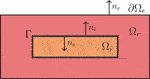FIGURE 1. Illustration of a two-dimensional version of the domain for the EMI model for a single cell. The domain consists of an intracellular domain, ${\text{Ω}}_{i}$, surrounded by an extracellular domain, ${\text{Ω}}_{e}$. The membrane, $\text{Γ}$, is defined as the boundary between the intracellular and extracellular domains. The figure is reused from Ref. 47 with permission from the publisher.

FIGURE 2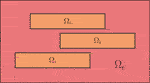FIGURE 2. Illustration of the EMI model domain for a collection of isolated cells. The spatial splitting approach described in Section 2.2 for a single cell may also be applied for this type of cell collection.

### 2.1. The EMI Model

In the EMI model for a single cell, like illustrated in Figure 1, we consider an intracellular domain, ${\text{Ω}}_{i}$, surrounded by an extracellular domain, ${\text{Ω}}_{e}$. The boundary between the intracellular and extracellular domains defines the cell membrane, $\text{Γ}$. The EMI model (see, e.g., Ref. 8) describes the electric potential in these domains and is given by the following system of equations:

$∇⋅σe∇ue=0 in Ωe,(1)$
$∇⋅σi∇ui=0 in Ωi,(2)$
$ue=0 at ∂ΩeD,(3)$
$ne⋅σe∇ue=0 at ∂ΩeN,(4)$
$ne⋅σe∇ue=−ni⋅σi∇ui≡Im at Γ,(5)$
$ui−ue=v at Γ,(6)$
$vt=1Cm(Im−Iion) at Γ,(7)$
$st=F at Γ.(8)$

Here, ${u}_{e}$ is the extracellular potential defined in ${\text{Ω}}_{e}$, ${u}_{i}$ is the intracellular potential defined in ${\text{Ω}}_{i}$, and v is the membrane potential defined at $\text{Γ}$. The potentials are all given in units of mV. The parameters ${\sigma }_{e}$ and ${\sigma }_{i}$ represent the extracellular and intracellular conductivites, respectively, and are given in units of mS/cm. Furthermore, ${n}_{e}$ and ${n}_{i}$ denote the outward pointing unit normal vectors of the extracellular and intracellular spaces, respectively, and ${C}_{m}$ represents the specific membrane capacitance, given in units of μF/cm2. Time is given in units of ms and length is given in units of cm.

The current density ${I}_{\text{ion}}$ represents the sum of the ionic current densities through different types of ion channels, pumps and exchangers on the cell membrane, and ${I}_{m}$ represents the sum ${I}_{\text{ion}}$ and the capacitive current density ${C}_{m}{v}_{t}$. All current densities are given in units of μA/cm2. The model for ${I}_{\text{ion}}$ typically involves a set of additional state variables describing the gating mechanisms of the ion channels and ionic concentrations. These variables are defined on $\text{Γ}$ and are denoted by s in the EMI system defined above. The dynamics of s are governed by a set of ordinary differential equations described by $F\left(v,s\right)$. In the computations of this paper, we let ${I}_{\text{ion}}$ and $F\left(v,s\right)$ be given by the Grandi et al. model for human ventricular cardiomyocytes .

On the outer boundary of the extracellular space, we apply the homogeneous Dirichlet boundary condition Eq. 3 on a part of the boundary, $\partial {\text{Ω}}_{e}^{D}$, and the homogeneous Neumann boundary condition Eq. 4 on the remaining part, $\partial {\text{Ω}}_{e}^{N}$. In the computations reported below, we apply Dirichlet boundary conditions on the outer extracellular boundary in the x- and y-directions and Neumann boundary conditions on the extracellular boundary in the z-direction.

### 2.2. The Coupled Discrete Model

In order to illustrate how the linear part of the EMI model Eqs 18 can be discretized and solved in a coupled manner, we describe below how to set up a finite difference discretization of the full EMI system. However, because of the possibly nonlinear terms included in F and ${I}_{\text{ion}}$, we use a temporal operator splitting method to split the EMI system into a linear part and a nonlinear part. The discrete version of this approach is taken from Ref. 8 but repeated here for completeness.

#### 2.2.1. Spatial and Temporal Splitting

The challenge we address in the present paper is to split the spatial coupling of the EMI model such that a solution algorithm can be founded on well-studied equations. In addition, we use temporal splitting of Eq. 7.

#### 2.2.2. Step 1: Membrane Dynamics

In the discrete model, we seek discrete approximations ${u}_{i}^{n,j,q,r}$, ${u}_{e}^{n,j,q,r}$, ${v}^{n,j,q,r}$, ${s}^{n,j,q,r}$ of the solutions at $t={t}_{n}=n\text{Δ}t$, $x={x}_{j}=j\text{Δ}x$, $y={y}_{q}=q\text{Δ}y$, and $z={z}_{r}=r\text{Δ}z$. It is important to notice that the approximations of v and s are only sought on $\text{Γ}$, the approximation of ${u}_{i}$ in ${\text{Ω}}_{i}$, and that of ${u}_{e}$ in ${\text{Ω}}_{e}$. For each time step ${t}_{n}$, we assume that the solutions ${v}^{n-1}$ and ${s}^{n-1}$ are known for the previous time step $\left(t={t}_{n-1}\right)$. In the first step of the temporal operator splitting procedure, we update the solution of v and s by solving

$vt=−1CmIion,(9)$
$st=F(v,s)(10)$

for a time step of $\text{Δ}t$ from the previous solutions ${v}^{n-1}$ and ${s}^{n-1}$. We let the solution of s from this step define the approximation of s at the current time step, ${s}^{n}$, and let the solution of v be denoted by $\overline{v}$. In the computations reported below, we solve Eqs 9 and 10 by taking m forward Euler steps of size $\text{Δ}{t}^{\text{*}}=\text{Δ}t/m$. We found the forward Euler scheme to produce stable solutions at $\text{Δ}{t}^{\text{*}}=0.001$ ms with the Grandi et al. model. If a stiffer cell model was used, a first-order generalized Rush–Larsen scheme  could be a more fitting choice, as it generally allows for a larger time step than forward Euler .

#### 2.2.3. Step 2: Coupled Linear EMI System

In the next step of the temporal operator splitting procedure, the remaining EMI system Eqs 17 with ${I}_{\text{ion}}=0$ is solved using a coupled finite difference discretization. A two-dimensional version of the computational grid used in this discretization is illustrated in Figure 3A. We observe that the computational grid consists of three types of nodes; extracellular nodes marked by $×$, intracellular nodes marked by ○, and membrane nodes marked by $\otimes$.

FIGURE 3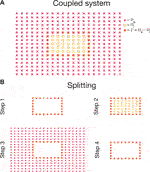FIGURE 3. Illustration of the computational mesh used in the coupled (A) and split (B) versions of the finite difference discretization of the EMI model. The mesh consists of three types of nodes; extracellular nodes marked by ×, intracellular nodes marked by ○, and membrane nodes marked by ⊗.

For the intracellular nodes, there is one unknown, ${u}_{i}$, and one governing equation (Eq. 2). This equation is discretized using the finite difference approximation

$σiΔx2(uin,j+1,q,r−2uin,j,q,r+uin,j−1,q,r)$
$+σiΔy2(uin,j,q+1,r−2uin,j,q,r+uin,j,q−1,r)(11)$
$+σiΔz2(uin,j,q,r+1−2uin,j,q,r+uin,j,q,r−1)=0.$

Likewise, for the majority of the extracellular nodes, there is one unknown, ${u}_{e}$, and one governing equation (Eq. 1), which is discretized using Eq. 11 with ${\sigma }_{i}$ and ${u}_{i}$ replaced by ${\sigma }_{e}$ and ${u}_{e}$, respectively. For the extracellular nodes on $\partial {\text{Ω}}_{e}^{D}$, Eq. 11 is replaced by the discrete version of Eq. 3$\left({u}_{e}^{n,j,q,r}=0\right)$. Furthermore, for extracellular nodes on $\partial {\text{Ω}}_{e}^{N}$ (i.e., for the boundary in the z-direction), the finite difference approximation

$uen,j,q,r+1−uen,j,q,r−12Δx=0(12)$

of Eq. 4 is used to find substitutions for either ${u}_{e}^{n,j,q,r+1}$ or ${u}_{i}^{n,j,q,r-1}$ located outside the computational domain in the extracellular version of Eq. 11.

For the nodes located at the membrane, there are three unknowns, ${u}_{i}$, ${u}_{e}$, v, and three governing equations (Eqs 57). For the flux equality equation, Eq. 5, we use a standard first-order finite difference approximation, and for Eq. 6, we use the discrete version ${v}^{n,j,q,r}={u}_{i}^{n,j,q,r}-{u}_{e}^{n,j,q,r}$. For Eq. 7 with ${I}_{\text{ion}}=0$, we use an implicit time discretization

$vn,j,q,r−v¯j,q,rΔt=1CmImn,j,q,r,(13)$

where ${I}_{m}^{n,j,q,r}$ is computed using the same finite differences as for Eq. 5 and ${\overline{v}}^{j,q,r}$ is the solution of v from Step 1 of the operator splitting procedure. Note that for membrane nodes located at the lines where two membrane planes intersect, we compute two versions of ${I}_{m}^{n,j,q,r}$, one for the normal derivative of each of the membrane planes and define ${I}_{m}^{n,j,q,r}$ as the mean value of these two versions. For corner nodes where three membrane planes intersect, we similarly compute three versions of ${I}_{m}^{n,j,q,r}$ and define ${I}_{m}^{n,j,q,r}$ as the mean value of these three versions.

Collecting the equations of the discrete version of the linear part of the EMI model, we end up with a spatially coupled system of equations consisting of one equation for every computational node plus two extra equations for each membrane node.

### 2.3. Robin/Neumann Spatial Splitting of the EMI Model

The coupled discrete version of the EMI model may be used to find accurate solutions of the system (see, e.g., Refs 810). However, in some cases it would be much more convenient to be able to rewrite the EMI system as a set of more standard problems that can be solved individually using existing well-developed solution methods for such standard problems. Linear systems arising from discretizations of elliptic partial differential equations have been under intense scrutiny for decades and the efforts have resulted in optimal solution strategies; see, e.g., Ref. 30. Our aim is therefore to introduce a spatial operator splitting algorithm for the EMI model that results in sub-problems formulated in terms of standard elliptic problems, thus enabling the use of optimal solvers.

In this section, we describe a method for constructing such a splitting of the EMI model. In this approach, for each time step, we will solve the equations of the intracellular and extracellular spaces separately as standard Laplace equations with Robin, Neumann or Dirichlet boundary conditions on the boundary of the domains. The algorithm described in this section is summarized in Algorithm 1.

#### 2.3.1. Step 1: Membrane Dynamics

In the first step of the splitting procedure, we solve the nonlinear part of the EMI model just like in the coupled discrete model. In other words, we update the solution of the membrane potential, v, and the remaining membrane state variables, s, by solving the system of ordinary differential equations

$vt=−1CmIion,(14)$
$st=F(v,s)(15)$

at $\text{Γ}$ for one time step with the solutions from the previous time step ${v}^{n-1},{s}^{n-1}$ as initial conditions. We let the solution of s from this step define the approximation of s at the current time step, ${s}^{n}$, and let the solution of v be denoted by $\overline{v}$.

#### 2.3.2. Step 2: Intracellular System

In the next step of the approach, we find an approximation of the intracellular potential, ${u}_{i}^{n}$, by solving Eq. 2 and using Eqs. 6, 7 (with ${I}_{\text{ion}}=0$ and initial condition $\overline{v}$) as boundary conditions for the membrane. In Eq. 6, we let ${u}_{e}^{n}$ be approximated by ${\overline{u}}_{e}$ computed as follows: In the first iteration of Step 2, we let ${\overline{u}}_{e}={u}_{e}^{n-1}$, and in the first time step, we assume that at $t=0$, we have ${u}_{e}^{0}=0$. For the next iterations, the approximation ${\overline{u}}_{e}$ is taken from the solution of the previous iteration of the splitting algorithm. Inserting the approximation of ${u}_{e}^{n}$ into Eq. 6, we get

$vn≈uin−u¯e.(16)$

In Eq. 7, we discretize the time derivative using the implicit approximation

$vn−v¯Δt=1CmImn.$

Inserting Eq. 16 yields

$uin−u¯e−v¯≈ΔtCmImn,$

and inserting the definition of ${I}_{m}$ from Eq. 5, we obtain

$uin+ΔtCmni⋅σi∇uin≈v¯+u¯e.$

This defines a Robin boundary condition at $\text{Γ}$ for the intracellular domain. Summarizing, Step 2 of the splitting procedure consists of solving

$∇⋅σi∇u¯i=0 in Ωi,(17)$
$u¯i+ΔtCmni⋅σi∇u¯i=v¯+u¯e at Γ,(18)$

to find an approximation of ${u}_{i}^{n}$, denoted by ${\overline{u}}_{i}$.

#### 2.3.3. Step 3: Extracellular System

In the third step of the procedure, we update the extracellular potential by solving Eq. 1 with boundary conditions given by Eqs 35. Here, ${n}_{i}\cdot {\sigma }_{i}\nabla {u}_{i}$ in Eq. 5 is found from the solution of Step 2. In other words, Step 3 consists of computing

$I¯m=−ni⋅σi∇u¯i(19)$

from the solution of ${\overline{u}}_{i}$ from Step 2, and then solving

$∇⋅σe∇u¯e=0 in Ωe,(20)$
$u¯e=0 at ∂ΩeD,(21)$
$ne⋅σe∇u¯e=0 at ∂ΩeN,(22)$
$ne⋅σe∇u¯e=I¯m at Γ,(23)$

to find an approximation ${\overline{u}}_{e}$ of ${u}_{e}^{n}$.

#### 2.3.4. Iteration for the Intracellular-Extracellular Coupling

After solving Step 2 and Step 3 once, we obtain a new approximation ${\overline{u}}_{e}$ for ${u}_{e}^{n}$. This approximation may be used to define a new approximation given by Eq. 16, and Step 2 and Step 3 may be repeated to obtain more accurate estimates of ${\overline{u}}_{i}$ and ${\overline{u}}_{e}$ for ${u}_{i}^{n}$ and ${u}_{e}^{n}$, respectively. After repeating these steps for a number of iterations, ${N}_{\text{it}}$, we define

$uin=u¯i,(24)$
$uen=u¯e.(25)$

#### 2.3.5. Step 4: Updating the Membrane Potential

In the final step of the procedure, the membrane potential, v, is updated using the solutions of ${u}_{i}$ and ${u}_{e}$ from the final iteration of Step 2 and Step 3. We use Eq. 6 and set

$vn=uin−uen at Γ.(26)$

### 2.4. Discrete Version of the Splitting Approach

An illustration of the computational mesh used in each of the steps of the finite difference discretization of the splitting method is found in Figure 3B. In the discrete version of the splitting approach, we solve Step 1 just like for the coupled version by taking m forward Euler steps of size $\text{Δ}{t}^{\text{*}}=\text{Δ}t/m$. In Step 2, we consider the nodes representing the membrane and the purely intracellular points and we have one equation and one unknown, ${\overline{u}}_{i}$, in all these points. For the purely intracellular points, we discretize Eq. 17 using Eq. 11. For the membrane points, we discretize ${n}_{i}\cdot {\sigma }_{i}\nabla {\overline{u}}_{i}$ in Eq. 18 using a standard first-order finite difference approximation. In Step 3, we discretize Eqs 2022 in the same manner as for the coupled system, and use standard first-order finite difference approximations of ${n}_{i}\cdot {\sigma }_{i}\nabla {\overline{u}}_{i}$ and ${n}_{e}\cdot {\sigma }_{e}\nabla {\overline{u}}_{e}$ in Eqs 19 and 23, respectively. In Step 4, we use the straightforward discrete version of Eq. 26.

### 2.5. Numerical Comparison of Solution Methods

In order to investigate the accuracy of the splitting method introduced above, we set up a simple test case with a single cell surrounded by an extracellular space. The cell is located in the center of the domain, and the size of the entire domain $\text{Ω}={\text{Ω}}_{i}\cup {\text{Ω}}_{e}$ is 180 μm $×$ 130 μm $×$ 26 μm. The default parameter values used in the simulation are given in Table 1, and the membrane dynamics are modeled by the epicardial version of the Grandi et al. model for human ventricular cardiomyocytes . In addition, 20% of the cell in the lower x-direction is stimulated by a membrane current of −40 μA/cm2.

TABLE 1
ALGORITHM 1

Figures 4 and 5 show a comparison of the solutions of the coupled approach and the splitting approach applied to this simple test problem. The left panel shows the extracellular potential, ${u}_{e}$, and the right panel shows the intracellular potential, ${u}_{i}$. Furthermore, the upper panel shows the solution of the coupled approach, the middle panel shows the solution of the splitting approach, and the lower panel shows the absolute value of the difference between the two solutions. In Figure 4 we use one iteration $\left({N}_{\text{it}}=1\right)$ in the splitting approach, and in Figure 5, we use five iterations $\left({N}_{\text{it}}=5\right)$. We observe that the coupled and splitting approaches result in very similar solutions for both ${u}_{e}$ and ${u}_{i}$ and for both one and five iterations of the splitting approach. However, the difference is reduced when five iterations are used compared to when one iteration is used. For ${N}_{\text{it}}=1$, the maximum difference between the two solutions is about ${10}^{-6}$ mV for ${u}_{e}$ and ${10}^{-2}$ mV for ${u}_{i}$. For ${N}_{\text{it}}=5$, the maximum difference is reduced to about ${10}^{-14}$ mV for ${u}_{e}$ and ${10}^{-10}$ mV for ${u}_{i}$.

FIGURE 4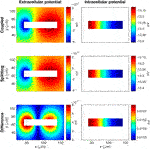FIGURE 4. Comparison of the solutions of the coupled (C) and splitting (S) approaches for the EMI model for a single cell. The left panel shows ue and the right panel shows ui . Furthermore, the upper panel shows the solution of the C approach, the middle panel shows the solution of the S approach, and the lower panel shows the absolute value of the difference between the two solutions. The solutions are collected at t = 3.5 ms, and the plots show the solutions in a sheet in the center of the domain in the z‐direction. The parameters used in the simulations are specified in Table 1, and we use Nit = 1 in the S approach.

FIGURE 5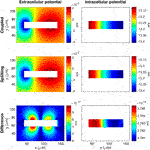FIGURE 5. Comparison of the solutions of the coupled and splitting approaches for the EMI model like in Figure 4, except that we use five iterations, Nit = 5, instead of one in the splitting approach.

In Table 2, we report the difference between the solutions of the two approaches in the form of the relative, discrete ${l}^{2}$-norm of the difference. We consider a number of different values of $\text{Δ}t$ for the splitting approach and compare these solutions to the solution of the coupled approach for $\text{Δ}t=0.001$ ms. In the splitting approach, we take five iterations for each time step $\left({N}_{\text{it}}=5\right)$. In the table, we observe that as $\text{Δ}t$ is reduced for the splitting approach, the difference between the two solutions decreases with a rate roughly equal to $\text{Δ}t$.

TABLE 2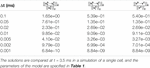TABLE 2. Difference between the solution of the coupled approach (${u}_{i}^{C}$, ${u}_{e}^{C}$, ${v}^{C}$) with $\text{Δ}t=0.001$ ms and the solution of the splitting approach (${u}_{i}^{S}$, ${u}_{e}^{S}$, ${v}^{S}$) for a number of different values of $\text{Δ}t$ and with ${N}_{\text{it}}=5$.

## 3. Connected Cells

As observed in the previous section, the proposed splitting method for the EMI model for a single cell appears to provide accurate solutions for reasonable time step sizes. However, in cases where cells are connected by gap junctions like illustrated in Figure 6, the splitting approach needs to be extended to account for the coupling between cells through the gap junctions. Again, the aim of the splitting is to obtain standard uncoupled Laplace problems in each domain and thereby allow solution of the originally coupled system by solving decoupled systems for the individual cells in parallel. In this section, we describe such an extension of the splitting method.

FIGURE 6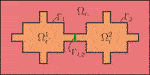FIGURE 6. Two-dimensional illustration of the EMI model domain for two connected cells. The domain consists of two cells, Ω1i and Ω2i , with cell membranes denoted by Γ1 and Γ2, respectively. The cells are connected to each other by the intercalated disc, Γ1,2, and surrounded by an extracellular space, denoted by Ωe. The figure is reused from Ref. 48 with permission from the publisher.

### 3.1. The EMI Model for Connected Cells

A two-dimensional version of the domain for the EMI model for two connected cells is illustrated in Figure 6. Two cells, ${\text{Ω}}_{i}^{1}$ and ${\text{Ω}}_{i}^{2}$, are surrounded by an extracellular space, ${\text{Ω}}_{e}$, and the boundary between ${\text{Ω}}_{e}$ and each of the cells ${\text{Ω}}_{i}^{1}$ and ${\text{Ω}}_{i}^{2}$ consists of the cell membranes denoted by ${\text{Γ}}_{1}$ and ${\text{Γ}}_{2}$, respectively. In addition, the boundary between the two intracellular domains defines an intercalated disc, denoted by ${\text{Γ}}_{1,2}$. The EMI model describes the electric potential in such a domain and is given by the equations

$∇⋅σe∇ue=0 in Ωe,(27)$
$∇⋅σi∇uik=0 in Ωik,(28)$
$ue=0 at ∂ΩeD,(29)$
$ne⋅σe∇ue=0 at ∂ΩeN,(30)$
$ne⋅σe∇ue=−nik⋅σi∇uik≡Imk at Γk,(31)$
$uik−ue=vk at Γk,(32)$
$vtk=1Cm(Imk−Iionk) at Γk,(33)$
$stk=Fk at Γk,(34)$
$nik˜⋅σi∇uik˜=−nik⋅σi∇uik≡Ik,k˜ at Γk,k˜,(35)$
$uik−uik˜=wk at Γk,k˜,(36)$
$wtk=1Cg(Ik,k˜−Igapk) at Γk,k˜,(37)$

for each cell k and each neighboring cell $\stackrel{˜}{k}$. Here, ${u}_{e}$, ${u}_{i}^{k}$, ${v}^{k}$ are the extracellular, intracellular and membrane potentials in ${\text{Ω}}_{e}$, ${\text{Ω}}_{i}^{k}$ and at ${\text{Γ}}^{k}$, respectively. In addition, ${w}^{k}={u}_{i}^{k}-{u}_{i}^{\stackrel{˜}{k}}$ denotes the potential difference at an intercalated disc, ${\text{Γ}}_{k,\stackrel{˜}{k}}$. Note here that at a given intercalated disc, this potential difference is defined differently for the two adjoining cells. For instance, at the intercalated disc ${\text{Γ}}_{1,2}$ illustrated in Figure 6, ${w}^{1}={u}_{i}^{1}-{u}_{i}^{2}$ and ${w}^{2}={u}_{i}^{2}-{u}_{i}^{1}$. The current density ${I}_{\text{gap}}^{k}$ (in μA/${\text{cm}}^{2}$) represents the ionic current density through the gap junctions at the intercalated disc and is given by

$Igapk=1Rgwk,(38)$

where ${R}_{g}$ is the gap junction resistance (in k$\text{Ω}$${\text{cm}}^{2}$), and ${C}_{g}$ in Eq. 37 represents the specific capacitance of the intercalated disc. Furthermore, ${I}_{k,\stackrel{˜}{k}}$ (in μA/${\text{cm}}^{2}$) represents the sum of ${I}_{\text{gap}}^{k}$ and the capacitive current over the gap junction, ${C}_{g}{w}_{t}^{k}$. For definitions and units for the remaining variables and parameters, see Section 2.1.

### 3.2. Discrete Coupled Version of the Model for Connected Cells

A two-dimensional version of the computational mesh used in the finite difference discretization of the EMI model for two connected cells is illustrated in Figure 7A. We observe that in this case, there are four different types of nodes; nodes located in the extracellular space, marked by $×$, nodes located in the intracellular space, marked by ○, nodes located at the membrane, marked by $\otimes$, and, finally, nodes located at the intercalated disc, marked by $•$.

FIGURE 7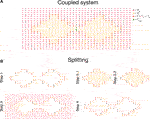FIGURE 7. Illustration of the computational mesh used in the coupled (A) and split (B) versions of the finite difference discretization of the EMI model for two connected cells. The mesh consists of four types of computational nodes; nodes located in the extracellular domain, marked by $×$, nodes located in the intracellular domain, marked by °, nodes located at the membrane, marked by $\otimes$, and nodes located at the intercalated disc, marked by $•$.

In the coupled discrete version of the EMI model for connected cells, we split the EMI model into a nonlinear part and a linear part using the same temporal operator splitting procedure as for the discrete coupled model for a single cell (see Section 2.2). Moreover, the first nonlinear step, solving the ordinary differential equations describing the membrane dynamics, is solved in exactly the same manner as for the single cell case (see Section 2.2.2).

Furthermore, in the finite difference discretization of the linear part of the EMI model we use the exact same approach to discretize the equations for the intracellular, extracellular and membrane nodes as for the single cell case (see Section 2.2.3). The only exceptions requiring special attention in the case of connected cells are the extracellular nodes located directly below or above the intercalated disc (see Figure 7A). For these nodes, we enforce a no-flow boundary condition and set the extracellular potential equal to the potential in the purely extracellular nodes located directly above or below the corner nodes.

The nodes located at the intercalated disc are treated in exactly the same manner as the membrane nodes except that ${I}_{\text{gap}}^{k}$ is not set to zero in Eq. 37. We discretize ${n}_{i}^{\stackrel{˜}{k}}\cdot {\sigma }_{i}\nabla {u}_{i}^{\stackrel{˜}{k}}$ and ${n}_{i}^{k}\cdot {\sigma }_{i}\nabla {u}_{i}^{k}$ in Eq. 35 using standard first-order finite differences, and use a straightforward discrete version of Eq. 36. Finally, Eq. 37 is discretized in time using an implicit finite difference approximation

$wk,n−wk,n−1Δt=1Cg(Ik,k˜n−1Rgwk,n),$

where a first-order finite difference approximation of $-{n}_{i}^{k}\cdot {\sigma }_{i}\nabla {u}_{i}^{k,n}$ is used to define ${I}_{k,\stackrel{˜}{k}}^{n}$.

### 3.3. Splitting Approach for the EMI Model for Connected Cells

We now want to split the intracellular subdomains in order to obtain classical elliptic equations defined on small domains. In this splitting approach, the linear part of the EMI model is for each time step split into standard Laplace problems with Robin, Neumann or Dirichlet boundary conditions. These problems can be solved separately in each of the domains ${\text{Ω}}_{i}^{k}$ and ${\text{Ω}}_{e}$.

Steps 1, 3, and 4 of the splitting approach are exactly the same for connected cells as for the single cell case (see Section 2.3), but Step 2 is extended to account for the cell coupling at the intercalated discs, ${\text{Γ}}_{k,\stackrel{˜}{k}}$. In this section we therefore only describe the extended version of Step 2 and refer to Section 2.3 for descriptions of the remaining steps of the procedure. In addition, the full splitting algorithm for coupled cells is summarized in Algorithm 2.

#### 3.3.1. Step 2: Intracellular System

In Step 2 of the splitting approach for connected cells, we update the intracellular potential by solving Eq. 28 in each of the intracellular domains, ${\text{Ω}}_{i}^{k}$. On the membrane, ${\text{Γ}}_{k}$, we define Robin boundary conditions from Eqs 32 and 33, just like in the single cell case (see Section 2.3.2). What remains is to define a boundary condition for the intercalated disc, ${\text{Γ}}_{k,\stackrel{˜}{k}}$, from Eqs 3537.

We define this boundary condition in an iterative manner, where we for each iteration use an approximation of ${w}^{k,n}$, denoted by ${\overline{w}}^{k}$. In the first iteration, we set ${\overline{w}}^{k}={w}^{k,n-1}$. Discretizing the time derivative in Eq. 37 using an implicit finite difference and inserting Eq. 38, we obtain

$Ik,k˜n=1Rgwk,n+Cgwk,n−wk,n−1Δt.$

Replacing ${w}^{k,n}$ by the approximation ${\overline{w}}^{k}$, we get

$Ik,k˜n≈1Rgw¯k+Cgw¯k−wk,n−1Δt.$

This defines an approximation of the Neumann boundary condition (Eq. 35) for ${\text{Ω}}_{i}^{k}$. In each iteration of the intracellular system, we therefore solve

$∇⋅σi∇u¯ik=0 in Ωik,(39)$
$u¯ik+ΔtCmnik⋅σi∇u¯ik=v¯k+u¯e at Γk,(40)$
$−nik⋅σi∇u¯ik=1Rgw¯k+Cgw¯k−wk,n−1Δt at Γk,k˜,(41)$

for each cell k to find temporary approximations of ${u}_{i}^{k,n}$, denoted by ${\overline{u}}_{i}^{k}$.1 Finally, we update ${\overline{w}}^{k}$ using Eq. 36 on the form

$w¯k=u¯ik−u¯ik˜.(42)$

This procedure (Eqs 3942) is continued for a number of iterations, denoted by ${M}_{\text{it}}$.

ALGORITHM 2

### 3.4. Discrete Version of the Splitting Approach

Figure 7B shows an illustration of the computational mesh used in each of the steps of the finite difference discretization of the splitting approach for connected cells. The discrete versions of steps 1, 3, and 4 of the procedure are set up in the same manner as for a single cell (see Section 2.4). For each cell and in each iteration of Step 2, we consider the nodes representing the intercalated disc, the membrane and the purely intracellular points, and we have one equation and one unknown, ${\overline{u}}_{i}^{k}$, in all these nodes. For the purely intracellular nodes, we discretize Eq. 39 using Eq. 11. For the membrane and intercalated disc nodes, we discretize ${n}_{i}\cdot {\sigma }_{i}\nabla {\overline{u}}_{i}^{k}$ in Eqs 40 and 41 using a standard first-order finite difference.

### 3.5. Numerical Comparison of Solution Methods

In order to investigate the accuracy of the splitting method for the EMI model for connected cells, we extend the simple test case introduced in Section 2.5 to include a collection of 5 $×$ 5 connected cells. We still use the parameters specified in Table 1, unless otherwise specified. In order to allow for two-dimensional cell connections, each cell is extended with additional cubes of smaller width in the x- and y-directions (see Figure 8). The sizes of these additional cubes are given in Table 3. The distance between the intracellular domain and the extracellular boundary is 12 μm in the x- and y-directions and 4 μm in the z-direction. Furthermore, we stimulate the 2 $×$ 2 cells in the lower left corner by a 1 ms-long stimulus current of $-40$ μA/${\text{cm}}^{2}$, and we consider the solution at $t=2.5$ ms.

FIGURE 8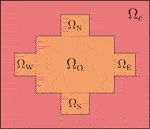FIGURE 8. Two-dimensional illustration of the geometry used for a single cell in the simulations of collections of connected cells. The intracellular domain is a composition of the subdomains ${\text{Ω}}_{\text{O}}$, ${\text{Ω}}_{\text{W}}$, ${\text{Ω}}_{\text{E}}$, ${\text{Ω}}_{\text{S}}$, and ${\text{Ω}}_{\text{N}}$, and all these subdomains are shaped as rectangular cuboids with sizes specified in Table 3. The figure is reused from Ref. 48 with permission from the publisher.

TABLE 3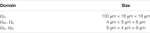TABLE 3. Specification of the size of the subdomains of a single cell used in the simulations of connected cells (see Figure 8).

Note that in the current implementation of the model, the intracellular and extracellular conductivities, ${\sigma }_{i}$ and ${\sigma }_{e}$, are assumed to be isotropic, and the anisotropy of cardiac tissue is represented by the anisotropic cell shape and distribution of gap junctions. Anisotropic conductivities, ${\sigma }_{i}$ and ${\sigma }_{e}$, could, however, also be straightforwardly incorporated in the model (see Eqs 2737). Using the parameters of the simple test case setup, the conduction velocity is approximately 26 cm/s in the longitudinal direction (x-direction) and approximately 6 cm/s in the transverse direction (y-direction).

In Figure 9, we compare the solutions of the coupled and splitting approaches applied to this test problem. In the splitting approach, we use five inner and outer iterations $\left({M}_{it}={N}_{it}=5\right)$. We observe that the coupled and splitting approaches result in very similar solutions for both ${u}_{e}$ and ${u}_{i}$. The maximum difference between the two solutions is about ${10}^{-8}$ mV for ${u}_{e}$ and ${10}^{-6}$ mV for ${u}_{i}$.

FIGURE 9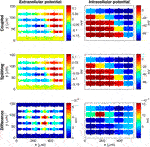FIGURE 9. Comparison of the solutions of the coupled (C) and splitting (S) approaches for the EMI model for connected cells. The left panel shows ${u}_{e}$ and the right panel shows ${u}_{i}$. Furthermore, the upper panel shows the solution of the C approach, the middle panel shows the solution of the S approach, and the lower panel shows the absolute value of the difference between the two solutions. The solutions are collected at $t=2.5$ ms, and the plots show the solutions in a sheet in the center of the domain in the z-direction. The parameters used in the simulations are specified in Tables 1 and 3, and we use five inner and outer iterations in the S approach $\left({M}_{\text{it}}={N}_{\text{it}}=5\right)$.

Table 4 compares the solution of the splitting approach for different values of $\text{Δ}t$ to the solution of the coupled approach using $\text{Δ}t=0.001$ ms. Again, we use five inner and outer iterations $\left({M}_{it}={N}_{it}=5\right)$ in the splitting approach. We observe that as the size of $\text{Δ}t$ is decreased for the splitting approach, the difference between the two solutions decreases with a rate roughly equal to $\text{Δ}t$. Note that in this case, $\text{Δ}t=0.1$ ms lead to instabilities for the splitting approach, so $\text{Δ}t=0.05$ ms is the smallest time step considered.

TABLE 4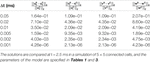TABLE 4. Difference between the solution of the coupled approach (${u}_{i}^{C}$, ${u}_{e}^{C}$, ${v}^{C}$, ${w}^{C}$) with $\text{Δ}t=0.001$ ms and the solution of the splitting approach (${u}_{i}^{S}$, ${u}_{e}^{S}$, ${v}^{S}$, ${w}^{S}$) for a number of different values of $\text{Δ}t$, using five inner and outer iterations $\left({N}_{it}={M}_{it}=5\right)$.

In Figure 10, we investigate how the absolute value of the difference between the intracellular potentials, computed using the two solution methods, depends on the time step, $\text{Δ}t$, the number of iterations used for the coupling between the intracellular and extracellular domains, ${N}_{\text{it}}$, and the number of iterations used for the coupling between the interior of the cells, ${M}_{\text{it}}$. In this case, we use the same $\text{Δ}t$ in the coupled and splitting approaches. In the first row of Figure 10, we observe that when only one inner and outer iteration is used in the splitting approach, the maximum difference between the solutions is in the range of 1–10 mV, and that the difference decreases as the time step is decreased. In the next rows, we observe that the difference between the solutions of the two methods decreases as we increase the number of iterations used in the splitting approach. For $\text{Δ}t=0.05$ ms, we see that the maximum difference is decreased from about 8 mV to about 1.5 mV if ${N}_{\text{it}}$ or ${M}_{\text{it}}$ is increased to five. Moreover, if both ${N}_{\text{it}}$ and ${M}_{\text{it}}$ is set to five, the maximum difference decreases to less than 0.01 mV. For $\text{Δ}t=0.01$ ms and $\text{Δ}t=0.001$ ms, we observe that the difference decreases more extensively when the number of outer iterations, ${N}_{\text{it}}$, is increased to five than when the number of inner iterations, ${M}_{\text{it}}$, is increased to five. Furthermore, when both are set to five the maximum difference is about ${10}^{-6}$ for $\text{Δ}t=0.01$ ms and ${10}^{-4}$ for $\text{Δ}t=0.001$ ms.

FIGURE 10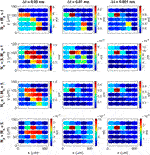FIGURE 10. Absolute values of the difference in ${u}_{i}$ computed using the coupled and splitting approaches for different combinations of the time step, $\text{Δ}t$, number of outer iterations, ${N}_{\text{it}}$, and number of inner iterations, ${M}_{\text{it}}$. The solutions are compared in the center of the domain in the z-direction at $t=2.5$ ms, and the parameters used in the simulations are specified in Tables 1 and 3.

Figure 11 shows a similar comparison of the solutions of the membrane potential, v, at the center of the membrane of the center cell as a function of time. We consider three different time steps $\text{Δ}t=0.05$ ms, $\text{Δ}t=0.01$ ms and $\text{Δ}t=0.001$ ms and some different combinations of the number of inner and outer iterations in the splitting algorithm. The upper panel of the figure shows the full upstroke of the action potential. In order to get a better impression of the differences between the solutions, the lower panel zooms in on the solution at the action potential peak. In the left panel, we observe that when only one iteration is used in the splitting approach $\left({N}_{it}={M}_{it}=1\right)$, there are clearly visible differences between the solutions of the splitting approach and the coupled approach. However, in the next panels, we observe that when the number of iterations is increased, the difference between the solutions decreases, and for ${N}_{\text{it}}={M}_{\text{it}}=5$, the solutions of the coupled and splitting approaches are completely indistinguishable in the plots.

FIGURE 11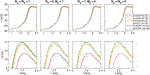FIGURE 11. Comparison of v at the center of the membrane of the center cell as a function of time computed using the coupled and splitting approaches for different combinations of the time step, $\text{Δ}t$, number of outer iterations, ${N}_{\text{it}}$, and number of inner iterations, ${M}_{\text{it}}$. The upper panel shows the full upstroke of the action potential, and the lower panel focuses on the action potential peak. The parameters used in the simulations are specified in Tables 1 and 3.

In Figure 12, we examine how the maximum difference in the computed intracellular potentials between the coupled and splitting approaches decreases as the number of iterations used in the splitting approach increases. In the left panel, we fix the number of inner iterations, ${M}_{\text{it}}$, at one or five and vary the number of outer iterations, ${N}_{\text{it}}$, between one and eight. We observe that the difference between the solutions of the two methods decreases as the number of outer iterations, ${N}_{\text{it}}$, increases. In addition, the difference is smaller if the number of inner iterations, ${M}_{\text{it}}$, is five than if it is one. In the right panel, we fix the number of outer iterations, ${N}_{\text{it}}$, at one or five and vary the number of inner iterations, ${M}_{\text{it}}$, between one and eight. In this case we observe that increasing ${M}_{\text{it}}$ above two or three when ${N}_{\text{it}}$ is fixed at one or five, respectively, does not seem to increase the accuracy of the splitting approach.

FIGURE 12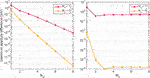FIGURE 12. Maximum absolute difference in ${u}_{i}$ at $t=2.5$ ms computed using the coupled and splitting approaches as the number of outer and inner iterations, ${N}_{\text{it}}$ and ${M}_{\text{it}}$, are increased. In the left panel, we increase the number of outer iterations, ${N}_{\text{it}}$, and consider the two cases ${M}_{\text{it}}=1$ and ${M}_{\text{it}}=5$. In the right panel, we increase the number of inner iterations, ${M}_{\text{it}}$, and consider the two cases ${N}_{\text{it}}=1$ and ${N}_{\text{it}}=5$. The parameters used in the simulations are specified in Tables 1 and 3.

## 4. Shared-Memory Parallel Implementation of the Splitting Algorithm

In this section, we will describe a parallel implementation of the splitting algorithm. The parallel implementation targets a hardware platform with a shared-memory system. Such parallel platforms are currently widely available, e.g., a server or desktop computer that has multiple sockets each with tens of CPU cores. The entire memory is composed of the memory domains that are directly connected to the sockets, allowing each CPU core to access any address in the whole memory space. The shared address space considerably eases the parallelization. However, care in programming is needed to maximize data locality, because accessing non-local parts of the memory is more costly than accessing the local part.

Important details of programming and performance enhancement will be discussed below, separately for the three decoupled numerical components of the splitting algorithm. An underlying principle is to use, whenever possible, existing software libraries for the different components. Such software libraries either use multiple threads to parallelize the computation internally, or are serially invoked inside a loop of parallel iterations.

### 4.1. The Intracellular Subdomains

Recall that the intracellular space consists of the individual excitable cells. Due to the splitting algorithm, the intracellular systems associated with the cells can be solved independently. This immediately brings a cell-level parallelism, because the individual intracellular systems can be assigned to the different CPU cores. With a spatial resolution of $\text{Δ}x=\text{Δ}y=\text{Δ}z=2\text{\hspace{0.17em}}\mu \text{m}$ there are about 5,300 degrees of freedom in the linear system for computing the intracellular potential of each cell. Such a small-size system suits well for a direct solver, see, e.g., Ref. 36, for which the LU factorization is an excellent choice. Moreover, since each intracellular matrix ${A}_{i}$ that arises from the finite difference discretization remains unchanged throughout an entire EMI simulation, the factorization step is executed only once. The resulting lower and upper triangular matrices, ${L}_{i}$ and ${U}_{i}$, are stored in memory, so that they are used in a forward-backward substitution procedure every time a linear system of the form ${A}_{i}x=b$ associated with an intracellular subdomain needs to be solved.

It is possible to adopt several threads to parallelize each forward-backward substitution step, but the amount of parallelism at this level is limited. Such a parallelization approach is thus not recommended unless the number of available CPU cores greatly exceeds the number of cells.

For computing the LU factorization and performing forward-back substitutions, we have chosen the serial version of the SuperLU library . We assign each of the linear systems ${A}_{i}x=b$ to an OpenMP thread so that multiple threads can work in parallel solving different linear systems. SuperLU was configured to use the included BLAS implementation, CBLAS, for the linear algebra routines. It is important that the BLAS implementation used by SuperLU is “thread safe” (i.e., that it supports being called from multiple threads) and does not attempt any parallelization of its own, as this interferes with the cell-level parallelization strategy employed here.

Apart from the aforementioned advantage that the LU factorization of each ${A}_{i}$ is only computed once, there is another opportunity for performance enhancement. This is true as long as we are interested in studying excitable cells of the same shape and size. Under such an assumption, the number of unique intracellular matrices is limited. More specifically, if the excitable cells form a rectangular 2D grid, then for each of the four sides, a cell can either be connected to a neighboring cell via a gap junction or not, leading to some slight changes in the cell’s geometry and boundary condition. As such, there are at most ${2}^{4}=16$ unique matrices, and in the examples in this paper where the cells form an $M×N$ rectangular grid with $M,N\ge 3$, there are only 9 different matrices (one for each of the four corners, one for each of the four sides, and one for the inner cells). To save memory usage, we can thus group together the linear systems sharing the same matrix ${A}_{i}$, and instead solve ${A}_{i}X=B$, where X and B are matrices that contain, respectively, all the relevant solution vectors and right-hand sides. Furthermore, we only need to compute and store the LU factorization for each of the 9 unique matrices. In order to ensure good data locality, the CPU cores that are assigned to the same intracellular matrix ${A}_{i}$ should preferably have direct access to the memory domain where ${L}_{i}$ and ${U}_{i}$ reside.

One remark is in order here. Although we discuss in this paper a two dimensional collection of cells, it is important to keep in mind that all the cell geometries are in 3D and all cells are surrounded by a 3D extracellular space. In fact, the EMI model for a mesh of cells can only be applied in 3D because in 2D the extracellular space would become disconnected.

### 4.2. The Extracellular Domain

The splitting algorithm requires the solution of a 3D Laplace equation for the entire extracellular domain with Neumann boundary conditions on the cell membranes. With a careful treatment of the boundary conditions, the finite difference discretization produces a symmetric and positive-definite matrix for the linear system associated with the extracellular domain. These properties of the matrix permit the use of a conjugate gradient (CG) iterative solver with an algebraic multigrid (AMG) preconditioner to solve the linear system. Under optimal conditions, the CG+AMG solver should require a constant number of iterations, irrespective of the number of degrees of freedom in the linear system, resulting in a solution time that grows linearly with the size of the linear system .

We used the CG and AMG implementations provided by ViennaCL  in our simulations, as ViennaCL supports shared-memory parallelization via OpenMP. The termination criterion $|Ax-b|/|b|<{10}^{-5}$ was used. The coarsening method inside AMG was set to maximum independent set (MIS) , and the interpolation method was set to smoothed aggregation. Additionally, the Jacobi smoother weight was set to 0.85 as this value seemed to result in the lowest number of iterations.

### 4.3. The Membrane

On each mesh node along the membrane, the ODE system given in Step 1 of Algorithm 2 is solved with a forward Euler scheme using a substepping time step $\text{Δ}{t}^{\text{*}}=1\text{\hspace{0.17em}}\mu \text{s}$. We also implemented a first-order generalized Rush–Larsen (GRL1) scheme, and found this theoretically more stable scheme to take about twice the computing time of forward Euler. Since the forward Euler scheme produced stable solutions for all the numerical experiments reported in this paper, the GRL1 scheme was not used. Within each time step, the ODE model can be solved independently on each membrane node, hence there is ample parallelism. We used the Gotran code generator  to generate C code for solving a single step for both the forward Euler and GRL1 schemes. The auto-generated code was then augmented with OpenMP directives enabling shared-memory parallel execution.

When initializing the memory for the ODE-model’s state variables and parameters, we perform a so-called “first touch” to ensure that all CPU cores work on the parts of the memory that are located closest to the cores in terms of connectivity. This step is important for achieving proper scaling on modern servers where the system memory is typically split into multiple NUMA (non-uniform memory access) domains.

### 4.4. Hardware Platform Setup

Two hardware testbeds were used to measure the performance of our parallel solver that implements the splitting algorithm. The specifications are listed in Table 5. The first testbed (System A) is a dual-socket server housing two AMD EPYC 7601 32-core CPUs, each configured with 8-channel memory operating at 2666 MT/s. This server has 2 TiB of memory in total. The second testbed (System B) is a quad-socket server with four Intel Xeon Platinum 8,168 24-core CPUs, each configured with 6-channel memory operating at 2,666 MT/s. The total memory size is 384 GiB. Notably, System A has four NUMA domains per socket,2 whereas System B only has one NUMA domain per socket. Both systems support 2-way simultaneous multi-threading (SMT), presenting two logical processors to the operating system for each physical CPU core. Our hardware test systems are arguably in the high end of the present-day CPU server market and thus allow us to explore the limits of what can be achieved in a shared-memory environment. Simulations with an even larger number of cells, or a finer resolution inside each cell, will eventually require an enhanced parallel simulator capable of utilizing multiple nodes on a distributed-memory system. Speedup due to increasing the number of nodes used can be expected as long as the communication overhead does not exceed the computation time per node.

TABLE 5

Our solver is mainly written in the Python programming language, but the most performance-critical parts are executed in C code which we call from Python via the ctypes module. The resulting multi-threaded parallel solver permits rapid prototyping while also being reasonably performant. In the results presented below, we have set environment variables to impose a “thread binding” that fixes the mapping of OpenMP threads to the logical processors such that the same core will work on the same part of the memory for every time step. All logical processors were assigned one thread each, resulting in 128 threads for System A and 192 threads for System B.

### 4.5. Performance Results

The mesh resolution was set to $\text{Δ}x=\text{Δ}y=\text{Δ}z=2\text{\hspace{0.17em}}\mu \text{m}$, and we use ${N}_{\text{it}}={M}_{\text{it}}=2$ in the splitting algorithm. ${N}_{E}$ is the number of unknowns in the linear system for the extracellular domain. $I{t}_{\text{CG}}$ is the mean number of preconditioned CG iterations required for the extracellular system. The time spent solving each of the three domains (Extracellular, Membrane and Intracellular) is listed as well as the total across all domains. On the right side, the times are divided by the number of cells to demonstrate the asymptotic scaling.

Tables 6 and 7 show the time usage per time step needed for 16 cells up to 65,536 and 16,348 cells for Systems A and B, respectively (The memory size on System B is not large enough to simulate $256×256$ cells.) More specifically, the tables report the average time usage per time step for time steps 6–10 in the simulation. As the number of cells grows, we can see the solution time per cell converging toward a constant. The number of AMG-preconditioned CG iterations for the extracellular system grows by about 71% while the number of unknowns increases by more than a factor of 2,800. System B outperforms System A for all the cases, which can be attributed to it having 50% higher theoretical memory bandwidth, 50% more cores, and higher clock speed than System A. Additionally, architectural differences between the CPUs could also have played a role.

TABLE 6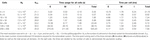TABLE 6. Time required to solve a time step of size $\text{Δ}t=0.02$ ms for geometries ranging from $4×4$ cells to $256×256$ cells using System A (see Section 4.4).

TABLE 7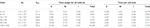TABLE 7. Time required to solve a time step of size $\text{Δ}t=0.02$ ms for geometries ranging from $4×4$ cells to $128×128$ cells using System B (see Section 4.4).

In Table 8, we consider the case of a 4 μm resolution for System A, and we are in this case able to perform simulations of up to 512 $×$ 256 cells. Note that in order to use a 4 μm resolution, the tissue geometry has been slightly altered. The distance from the cells to the extracellular boundary in the z-direction was extended to 8 μm, ${\text{Ω}}_{\text{O}}$ was of size 104 μm $×$ 16 μm $×$ 16 μm, and ${\text{Ω}}_{\text{W}}$, ${\text{Ω}}_{\text{E}}$, ${\text{Ω}}_{\text{S}}$, and ${\text{Ω}}_{\text{N}}$ had size 8 μm $×$ 8 μm $×$ 8 μm (see Figure 3).

TABLE 8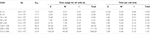TABLE 8. Time required to solve a time step of size $\text{Δ}t=0.02$ ms for geometries ranging from $4×4$ cells to $512×256$ cells using System A (see Section 4.4).

The mesh resolution was set to $\text{Δ}x=\text{Δ}y=\text{Δ}z=4\text{\hspace{0.17em}}\mu \text{m}$, and we use ${N}_{\text{it}}={M}_{\text{it}}=2$ in the splitting algorithm. ${N}_{E}$ is the number of unknowns in the linear system for the extracellular domain. $I{t}_{\text{CG}}$ is the mean number of preconditioned CG iterations required for the extracellular system. The time spent solving each of the three domains (Extracellular, Membrane and Intracellular) is listed as well as the total across all domains. On the right side, the times are divided by the number of cells to demonstrate the asymptotic scaling.

## 5. Discussion

Accurate modeling of excitable cells is important in order to understand how collections of such cells work. The EMI model provides a promising framework for such simulations, but it is challenging from a computational point of view. In this paper, we have developed an optimal splitting-based method that scales perfectly with the number of cells in the simulations. The main result of the paper is that we have been able to formulate a solution strategy that relies on intermediate steps that all are covered by standard theory in scientific computing.

We have seen in Table 8 that a collection of 512 $×$$256=131,072$ cells, with a mesh resolution of $\Delta x=4\text{\hspace{0.17em}}\text{μm}$, leads to linear systems with about 2.5$×{10}^{8}$ unknowns. In comparison, we found that the sinus node of the mouse heart would require about 3.5$×$${10}^{6}$ unknowns. Since the computing time scales linearly with the number of nodes, we estimate based on Table 8 that the sinus node of the mouse would require about 3 s of computing time per time step. A complete simulation of 300 ms would thus need about 24 hours of computing time (with Δt = 0.01 ms). This is a rough estimate of course, but it still illustrates that accurate simulation of physiologically interesting nodes is within reach.

The volume of the mouse ventricles is about 100 mm3 (see Ref. 42) or about 440 times larger than the mouse sinus node. In order to be able to simulate mouse ventricles within a 24-hour wall-clock time frame, we therefore would need a computer that is about 440 times faster than System A above, assuming that linear scaling would hold for even larger systems. This is actually well within today’s technological capability, because the world’s most powerful supercomputer at the time of writing, named Fugaku (see, e.g., Refs. 43 and 44), consists of 158,976 computers, each more powerful than System A (if we consider the available memory bandwidth). Although theoretically feasible, it remains to be seen whether a full action potential of a complete mouse heart can be simulated based on the EMI model.

The computing time may be reduced by applying adaptive time stepping and the number of nodes may be reduced by using an adaptive finite element method instead of the finite difference method. However, the finite difference scheme is exceptionally well suited for the computer systems A and B and fewer mesh points in the finite element method could therefore result in increased computing time per computational node (see Ref. 8). A possible path of future work can be to develop a distributed-memory parallel implementation of the splitting algorithm, so that the number of cells to be simulated is not limited by the computing speed and/or memory capacity of a single shared-memory platform.

A weakness of the current implementation is that, whereas the finite difference method is well suited for representing very simple geometries, it is less suited for complex geometrical shapes. For instance, representation of real geometries of the heart, and also including the fiber orientation of the heart muscle is necessary in many applications; see, e.g., Refs. 1, 45 and 46. In order to analyze problems with complex geometries using the EMI model, we will need to use the finite element method. Also, representing realistic geometries of individual cells is very challenging using the finite difference method, but will be easier using the finite element method. A finite element method based on the operator splitting scheme derived in the present report is currently under development. This code will clearly offer greater flexibility with respect to geometries, but the linear systems are less structured and therefore harder to solve in an optimal manner.

## 6. Conclusion

We have introduced a splitting scheme for the linear part of the EMI equations that allows us to use well developed theory for solving linear systems arising from the discretization of linear, elliptic partial differential equations. The resulting method is stable and is optimal in the sense that the CPU efforts scale linearly with the number of computational nodes. The method therefore enables simulations using far larger computing facilities than has been available in the present work.

## Data Availability Statement

The original contributions presented in the study are included in the article/Supplementary material, further inquiries can be directed to the corresponding author.

## Author Contributions

Operator splitting algorithm: KJ, AT. Parallelization: KH, XC. Programming: KJ, KH. Simulations: KJ, KH. All authors contributed to the writing of the paper.

## Funding

The research presented in this paper has benefited from the Experimental Infrastructure for Exploration of Exascale Computing (eX3), which is financially supported by the Research Council of Norway under contract 270053.

## Conflict of Interest

The authors declare that the research was conducted in the absence of any commercial or financial relationships that could be construed as a potential conflict of interest.

## Footnotes

1Note that in the first iteration of this procedure, the approximation of the capacitive current density, ${C}_{g}\frac{{\overline{w}}^{k}-{w}^{k,n-1}}{\text{Δ}t}$, is equal to zero because ${\overline{w}}^{k}={w}^{k,n-1}$.

2This stems from the CPU package being comprised of 4 dies, each with 8 cores and two memory channels.

## References

1. Franzone PC, Pavarino LF, Scacchi S. Mathematical cardiac electrophysiology. Cham, Switzerland: Springer International Publishing (2014). p. 397.

2. Sundnes J, Lines GT, Cai X, Nielsen BF, Mardal K-A, Tveito A. Computing the electrical activity of the heart. Heidelberg, Germany: Springer (2006). p. 318.

3. Whiteley JP. Physiology driven adaptivity for the numerical solution of the bidomain equations. Ann Biomed Eng (2007). 35(9):1510–20. doi:10.1007/s10439-007-9337-3

4. Mardal K-A, Nielsen BF, Cai X, Tveito A. An order optimal solver for the discretized bidomain equations. Numer Lin Algebra Appl (2007). 14(2):83–98. doi:10.1002/nla.501

5. Linge S, Sundnes J, Hanslien M, Lines GT, Tveito A. Numerical solution of the bidomain equations. Phil Trans Roy Soc Lond (1895). 367:1931–50. doi:10.1063/1.166300

6. Cervi J, Spiteri RJ. High-order operator splitting for the bidomain and monodomain models. SIAM J Sci Comput (2018). 40(2):A769–86. doi:10.1137/17m1137061

7. Ottino D, Scacchi S. Bpx preconditioners for the bidomain model of electrocardiology. J Comput Appl Math (2015). 285:151–68. doi:10.1016/j.cam.2015.02.011

8. Tveito A, Jæger KH, Kuchta M, Mardal K-A, Rognes ME. A cell-based framework for numerical modeling of electrical conduction in cardiac tissue. Front Phys (2017). 5:48. doi:10.3389/fphy.2017.00048

9. Jæger KH, Edwards AG, McCulloch A, Tveito A. Properties of cardiac conduction in a cell-based computationalmodel. PLoS Comput Biol (2019). 15(5):e1007042. doi:10.1371/journal.pcbi.1007042

10. Tveito A, Jæger KH, Lines GT, Paszkowski Ł, Sundnes J, Edwards AG, et al. An evaluation of the accuracy of classical models for computing the membrane potential and extracellular potential for neurons. Front Comput Neurosci (2017). 11:27. doi:10.3389/fncom.2017.00027

11. Hogues H, Leon LJ, Roberge FA. A model study of electric field interactions between cardiac myocytes. IEEE Trans Biomed Eng (1992). 39(12):1232–43. doi:10.1109/10.184699

12. Krassowska W, Neu JC. Response of a single cell to an external electric field. Biophys J (1994). 66(6):1768–76. doi:10.1016/s0006-3495(94)80971-3

13. Ying W, Henriquez CS. Hybrid finite element method for describing the electrical response of biological cells to applied fields. IEEE Trans Biomed Eng (2007). 54(4):611–20. doi:10.1109/tbme.2006.889172

14. Agudelo-Toro A, Neef A. Computationally efficient simulation of electrical activity at cell membranes interacting with self-generated and externally imposed electric fields. J Neural Eng (2013). 10(2):026019. doi:10.1088/1741-2560/10/2/026019

15. Roberts SF, Stinstra JG, Henriquez CS. Effect of nonuniform interstitial space properties on impulse propagation: a discrete multidomain model. Biophys J (2008). 95(8):3724–37. doi:10.1529/biophysj.108.137349

16. Stinstra JG, Henriquez CS, MacLeod RS. Comparison of microscopic and bidomain models of anisotropic conduction. In Computers in cardiology. Park City, UT: IEEE (2009). p. 657–60.

17. Stinstra J, MacLeod R, Henriquez C. Incorporating histology into a 3D microscopic computer model of myocardium to study propagation at a cellular level. Ann Biomed Eng (2010). 38(4):1399–414. doi:10.1007/s10439-009-9883-y

18. Stinstra JG, Roberts SF, Pormann JB, MacLeod RS, Henriquez CS. A model of 3D propagation in discrete cardiac tissue. In Computers in cardiology. Valencia, Spain: IEEE (2006). p. 41–44.

19. Stinstra JG, Hopenfeld B, MacLeod RS. On the passive cardiac conductivity. Ann Biomed Eng (2005). 33(12):1743–51. doi:10.1007/s10439-005-7257-7

20. Kucera JP, Rohr S, Rudy Y. Localization of sodium channels in intercalated disks modulates cardiac conduction. Circ Res (2002). 91(12):1176–82. doi:10.1161/01.res.0000046237.54156.0a

21. Tsumoto K, Ashihara T, Haraguchi R, Nakazawa K, Kurachi Y. Roles of subcellular Na + channel distributions in the mechanism of cardiac conduction. Biophys J (2011). 100(3):554–63. doi:10.1016/j.bpj.2010.12.3716

22. Louch WE, Sejersted OM, Swift F. There goes the neighborhood: pathological alterations in t-tubule morphology and consequences for cardiomyocyte Ca2+ handling. BioMed Res Int (2010). 2010:503906. doi:10.1155/2010/503906

23. Spach MS, Heidlage JF, Dolber PC, Barr RC. Electrophysiological effects of remodeling cardiac gap junctions and cell size. Circ Res (2000). 86(3):302–11. doi:10.1161/01.res.86.3.302

24. Veeraraghavan R, Salama ME, Poelzing S. Interstitial volume modulates the conduction velocity-gap junction relationship. Am J Physiol Heart Circ Physiol (2012). 302(1):H278–86. doi:10.1152/ajpheart.00868.2011

25. Veeraraghavan R, Gourdie RG, Poelzing S. Mechanisms of cardiac conduction: a history of revisions. Am J Physiol Heart Circ Physiol (2014). 306(5):H619–27. doi:10.1152/ajpheart.00760.2013

26. Niederer S, Mitchell L, Smith N, Plank G. Simulating human cardiac electrophysiology on clinical time-scales. Front Physiol (2011). 2(14):1–7. doi:10.3389/fphys.2011.00014

27. Csepe TA, Zhao J, Hansen BJ, Ning L, Lidiya VS, Lim P, et al. Human sinoatrial node structure: 3D microanatomy of sinoatrial conduction pathways. Prog Biophys Mol Biol (2016). 120(1–3):14–178. doi:10.1016/j.pbiomolbio.2015.12.011

28. Liu J, Noble PJ, Xiao G, Mohamed A, Dobrzynski H, Boyett MR, et al. Role of pacemaking current in cardiac nodes: insights from a comparative study of sinoatrial node and atrioventricular node. Prog Biophys Mol Biol (2008). 96(1–3):294–304. doi:10.1016/j.pbiomolbio.2007.07.009

29. Benzi M. Preconditioning techniques for large linear systems: a survey. J Comput Phys (2002). 182(2):418–77. doi:10.1006/jcph.2002.7176

30. Mele G, Ringh E, David E, Izzo F, Upadhyaya P, Elias J. Preconditioning for linear systems (2020).

31. Mardal K-A, Winther R. Preconditioning discretizations of systems of partial differential equations. Numer Lin Algebra Appl (2011). 18(1):1–40. doi:10.1002/nla.716

32. Wathen AJ. Preconditioning. Acta Numerica (2015). 24:329–76. doi:10.1017/s0962492915000021

33. Grandi E, Pasqualini FS, Bers DM. A novel computational model of the human ventricular action potential and Ca transient. J Mol Cell Cardiol (2010). 48:112–21. doi:10.1016/j.yjmcc.2009.09.019

34. Sundnes J, Artebrant R, Skavhaug O, Tveito A. A second-order algorithm for solving dynamic cell membrane equations. IEEE Trans Biomed Eng (2009). 56(10):2546–8. doi:10.1109/tbme.2009.2014739

35. Marsh ME, Ziaratgahi ST, Spiteri RJ. The secrets to the success of the Rush–Larsen method and its generalizations. IEEE Trans Biomed Eng (2012). 59(9):2506–15. doi:10.1109/TBME.2012.2205575

36. Bangerth W. Finite element methods in scientific computing Available from: https://www.math.colostate.edu/bangerth/videos/676/slides.34.pdf (Accessed July 1, 2020)..

37. Li XS. An overview of SuperLU. ACM Trans Math Software (2005). 31(3):302–25. doi:10.1145/1089014.1089017

38. Stüben K. A review of algebraic multigrid. J Comput Appl Math (2001). 128(1):281–309. doi:10.1016/s0377-0427(00)00516-1

39. Rupp K, Tillet P, Rudolf F, Weinbub J, Morhammer A, Grasser T, et al. ViennaCL—Linear algebra library for multi- and many-core architectures. SIAM J Sci Comput (2016). 38(5):S412–39. doi:10.1137/15m1026419

40. Bell N, Dalton S, Olson LN. Exposing fine-grained parallelism in algebraic multigrid methods. SIAM J Sci Comput (2012). 34(4):C123–52. doi:10.1137/110838844

41. Hake J, Finsberg H, Hustad KG, George B. Gotran–general ODE TRANslator Available from: https://github.com/ComputationalPhysiology/gotran (Accessed July 1, 2020).

42. Kaese S, Sander V. Cardiac electrophysiology in mice: a matter of size. Front Physiol (2012). 3:345. doi:10.3389/fphys.2012.00345

43.TOP500 Supercomputer Sites. Available from: https://www.top500.org/lists/2020/06/ (Accessed July 1, 2020).

44.Supercomputer Fugaku. Available from: https://www.fujitsu.com/global/about/innovation/fugaku/index.html (Accessed July 1, 2020).

45. Roth BJ, Beaudoin DL. Approximate analytical solutions of the bidomain equations for electrical stimulation of cardiac tissue with curving fibers. Phys Rev (2003). 67(5):051925. doi:10.1103/physreve.67.051925

46. Beaudoin DL, Roth BJ. The effect of the fiber curvature gradient on break excitation in cardiac tissue. Pacing clin electrophysiol (2006). 29(5):496–501. doi:10.1111/j.1540-8159.2006.00382.x

47. Jæger KH, Tveito A. Derivation of a Cell-Based Mathematical Model of Excitable Cells. In: Modeling excitable tissue. Cham: Springer. (2020). p. 1–13.

48. Jæger KH, Hustad KG, Cai X, Tveito A. Operator Splitting and Finite Difference Schemes for Solving the EMI Model. In: Modeling Excitable Tissue. Springer, Cham, (2020). p. 44–55.

Keywords: electrophysiological model, cardiac conduction, cell modeling, finite difference method, operator splitting algorithm

Citation: Jæger KH, Hustad KG, Cai X and Tveito A (2021) Efficient Numerical Solution of the EMI Model Representing the Extracellular Space (E), Cell Membrane (M) and Intracellular Space (I) of a Collection of Cardiac Cells. Front. Phys. 8:579461. doi: 10.3389/fphy.2020.579461

Received: 16 September 2020; Accepted: 27 October 2020;
Published: 13 January 2021.

Edited by:

Aljaz Godec, Max Planck Institute for Biophysical Chemistry, Germany

Reviewed by: